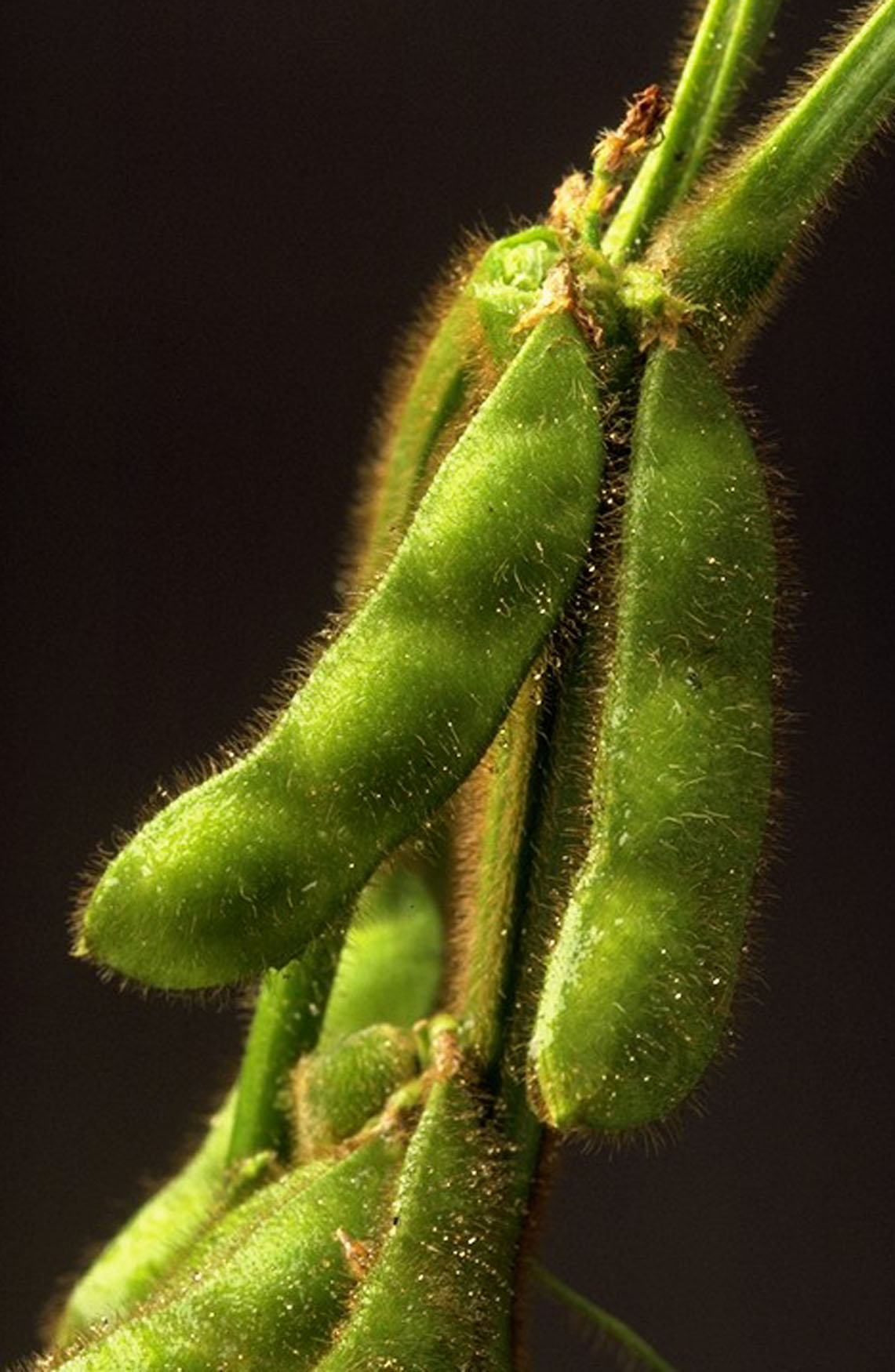August 19, 2015To estimate yield, four soybean yield components need to be considered: plants per acre, pods per plant, seeds per pod, and seeds per pound (seed size).  A printable worksheet to estimate soybean yield is available.  It is difficult to accurately predict soybean yield because of plant variability and fall weather conditions can influence seed size.  Estimates become more accurate as the growing season progresses.

To estimate soybean yield:

1. To calculate plants per acre, count the number of pod-bearing plants in 1/1,000th of an acre.  In 7.5-inch row spacing, count the number of plants in 69 feet, 8 inches of row.  In 15-inch row spacing, count the number of plants in 34 feet, 10 inches of row.  In 30-inch row spacing, count the number of plants in 17 feet, 5 inches of row.

2. To estimate pods per plant, count the number of pods (containing one or more seeds) from 10 plants selected at random.  Divide the total number of pods by 10 to get the average number of pods per plant.

3. To estimate the number of seeds per pod, count the number of seeds from 10 pods selected at random.  Generally, the number of seeds per pod is 2.5, but this number can be less in stressful environmental conditions.  Divide the total number of seeds by 10 to get the average number of seeds per pod.

4. To estimate the number of seeds per pound (seed size), assume that there are 3,000 seeds per pound.  If the soybean plants experienced stress, seed size will be reduced, and it will take more seeds to make one pound.  Use a seed size estimate of 3,500 seeds per pound if smaller seeds are expected because of late season stress.

Using the above estimates, the following formula is used to estimate soybean yield in bushels per acre:  bushels per acre = [(plants/1,000th acre) x (pods/plant) x (seeds/pod)] ÷ [(seeds/pound) x 0.06]

Read this on the OSU website.

2 methods for estimating corn yield